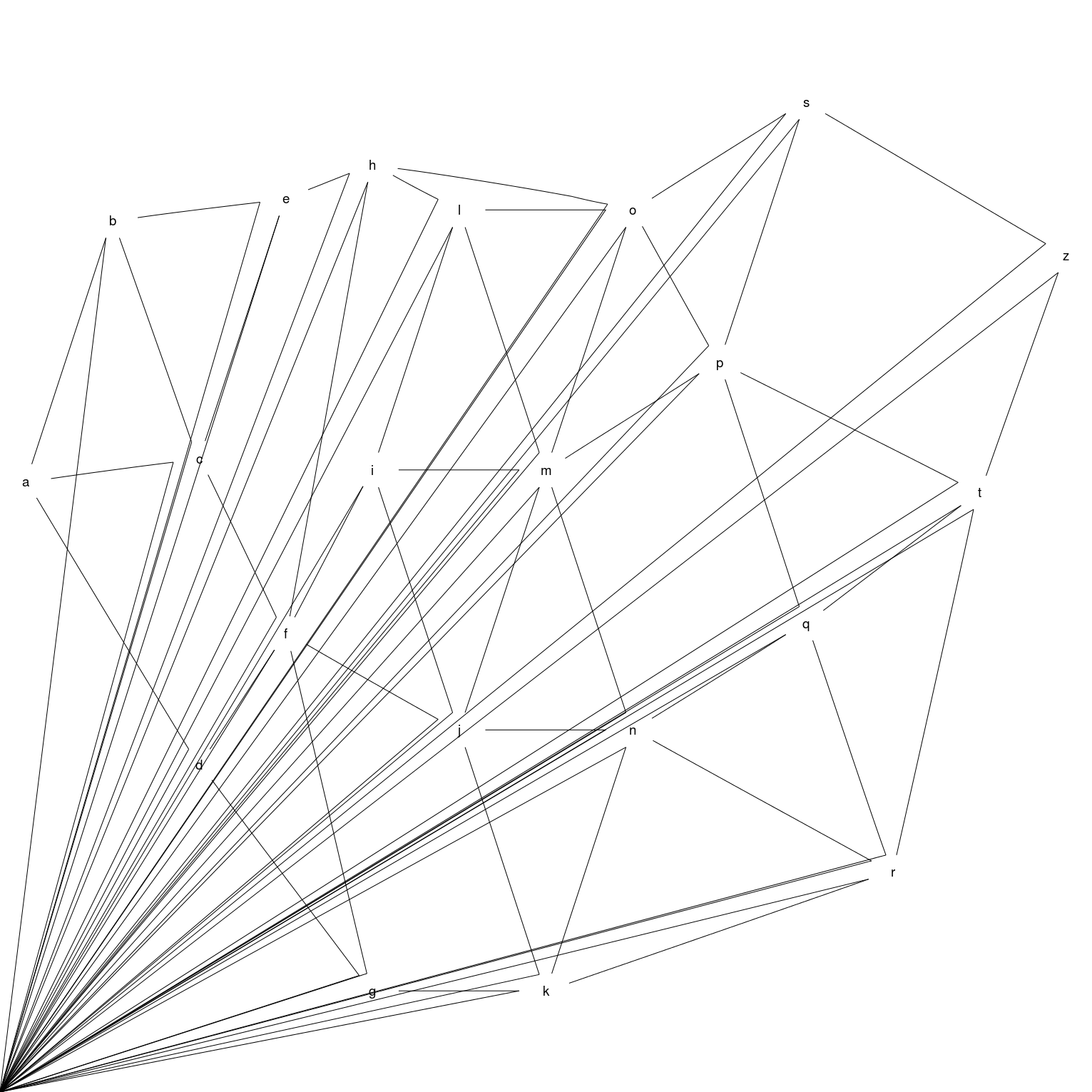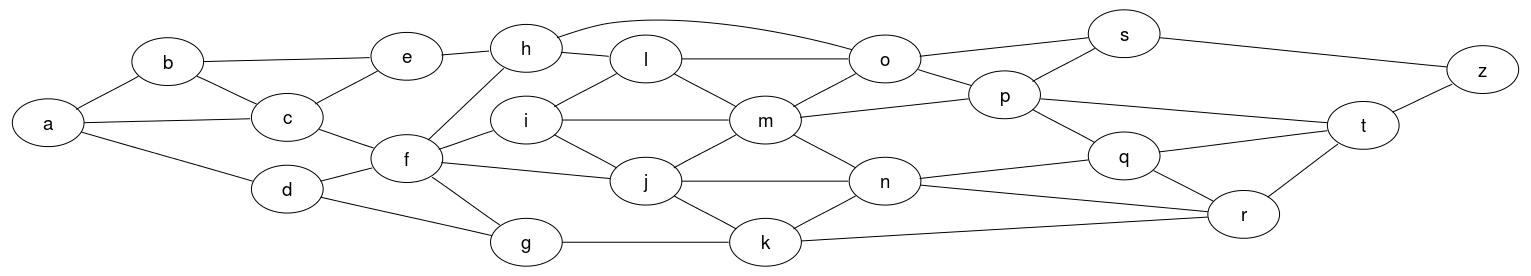# Example 8: large graph

An undirected graph with 21 nodes, 42 edges.

## Graph declaration

In all cases the graph is explicitly declared with ellipse shaped nodes. The dot file edges are declared in alphabetical order as this is the (assumed) default for how Rgraphviz will pass edges internally to graphviz.

 Dot file for graphviz: `````` graph { node [shape=ellipse]; rankdir=LR; // Left to Right, instead of Top to Bottom //nodes a b c d e f g h i j k l m n o p q r s t z; //edges a -- { b c d }; b -- { c e }; c -- { e f }; d -- { f g }; e -- h; f -- { h i j g }; g -- k; h -- { o l }; i -- { l m j }; j -- { m n k }; k -- { n r }; l -- { o m }; m -- { o p n }; n -- { q r }; o -- { s p }; p -- { s t q }; q -- { t r }; r -- t; s -- z; t -- z; } `````` R code for Rgraphviz and gridGraphviz: ```# load Rgraphviz library library(Rgraphviz) # nodes nodes <- c(letters[1:20], "z") # edges edgeList <- list(a=list(edges=c("b", "c", "d")), b=list(edges=c("a", "c", "e")), c=list(edges=c("a", "b", "e", "f")), d=list(edges=c("a", "f", "g")), e=list(edges=c("b", "c", "h")), f=list(edges=c("c", "d", "h", "i", "j", "g")), g=list(edges=c("d", "f", "k")), h=list(edges=c("e", "f", "o", "l")), i=list(edges=c("f", "l", "m", "j")), j=list(edges=c("f", "i", "m", "n", "k")), k=list(edges=c("g", "j", "n", "r")), l=list(edges=c("h", "i", "o", "m")), m=list(edges=c("i", "j", "l", "o", "p", "n")), n=list(edges=c("j", "k", "m", "q", "r")), o=list(edges=c("h", "l", "m", "s", "p")), p=list(edges=c("m", "o", "s", "t", "q")), q=list(edges=c("n", "p", "t", "r")), r=list(edges=c("k", "n", "q", "t")), s=list(edges=c("o", "p", "z")), t=list(edges=c("p", "q", "r", "z")), z=list(edges=c("t", "s"))) graph <- new("graphNEL", nodes=nodes, edgeL=edgeList, edgemode="undirected") ```

## Graph rendering:

### gridGraphviz (new)

``````>> dot -Tpng ex8.dot -o ex8.png
`````````# create Ragraph object
rag <- agopen(graph, "", attrs=list(node=list(shape="ellipse"),
graph=list(rankdir="LR")))
```
```#plot
plot(rag)
``````library(gridGraphviz)

# create Ragraph object
rag <- agopen(graph, "", attrs=list(node=list(shape="ellipse"),
graph=list(rankdir="LR")))
```
```#plot
grid.graph(rag)
```
```## Warning: Unsupported node shape; using 'box'
## Warning: Unsupported node shape; using 'box'
## Warning: Unsupported node shape; using 'box'
## Warning: Unsupported node shape; using 'box'
## Warning: Unsupported node shape; using 'box'
## Warning: Unsupported node shape; using 'box'
## Warning: Unsupported node shape; using 'box'
## Warning: Unsupported node shape; using 'box'
## Warning: Unsupported node shape; using 'box'
## Warning: Unsupported node shape; using 'box'
## Warning: Unsupported node shape; using 'box'
## Warning: Unsupported node shape; using 'box'
## Warning: Unsupported node shape; using 'box'
## Warning: Unsupported node shape; using 'box'
## Warning: Unsupported node shape; using 'box'
## Warning: Unsupported node shape; using 'box'
## Warning: Unsupported node shape; using 'box'
## Warning: Unsupported node shape; using 'box'
## Warning: Unsupported node shape; using 'box'
## Warning: Unsupported node shape; using 'box'
## Warning: Unsupported node shape; using 'box'
``````library(gridGraphviz)

# create Ragraph object
rag <- agopenTrue(graph, "",
attrs=list(node=list(shape="ellipse"),
graph=list(rankdir="LR")))
```
```#plot
grid.graph(rag)
```## Discussion

gridGraphviz (old) fails to produce ellipse shaped nodes, and produces "extra edges" which extend, in all cases, to the origin.